# xnaMugen Build 22343 (1/29) (Read 27410 times)

Started by FrantzX, January 30, 2009, 08:53:59 am

#### FrantzX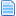##### Re: xnaMugen Build 22343 (1/29)
#21  February 07, 2009, 09:29:02 am
••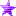These are the state controllers for the Rolling Buckler follow-ups:
[State 1000, ChangeState]
type = ChangeState
triggerall = Time > 4
trigger1 = Command = "x"
trigger2 = Var(50) = 1 || Var(50) = 2;NumTarget(5)
trigger2 = Command = "x"
value = 1025
ignorehitpause = 1
ctrl = 0

[State 1000, ChangeState]
type = ChangeState
triggerall = Time > 4
trigger1 = Command = "y"
trigger2 = Var(50) = 1 || Var(50) = 2;NumTarget(5)
trigger2 = Command = "y"
value = 1015
ignorehitpause = 1
ctrl = 0

[State 1000, ChangeState]
type = ChangeState
triggerall = Time > 4
trigger1 = Command = "z"
trigger2 = Var(50) = 1 || Var(50) = 2;NumTarget(5)
trigger2 = Command = "z"
value = 1005
ignorehitpause = 1
ctrl = 0

[State 1000, ChangeState]
type = ChangeState
triggerall = Time > 4
trigger1 = Command = "a"
trigger2 = Command = "b"
trigger3 = Command = "c"
trigger4 = Var(50) = 1 || Var(50) = 2;NumTarget(5)
trigger4 = Command = "a"  || Command = "b" || Command = "c"
value = 1035
ignorehitpause = 1
ctrl = 0

It looks to me that you should be able to transition to a follow up by pressing the correct button 5 ticks into the Rolling Buckler's state. Of course, the trigger "Var(50) = 1 || Var(50) = 2" makes it look as it you may have another coding issue.

---

This is in the command file. You are missing trigger3.
[State -1, Chain]
type = CtrlSet
triggerall = !Ctrl
trigger1 =  (StateNo = 52 || StateNo = 101 || StateNo = 106 || StateNo = 5120 || StateNo = 1305) && AnimTime = 0 ;Land States
trigger2 =  (StateNo = [200,499]) && AnimTime = 0 ;Standing and Crouching Basics
trigger4 = ((StateNo = 5201) || (StateNo = [900,999])) && AnimTime = 0 ;System States
trigger5 =   StateNo = 2800 && AnimTime = 0       ;Counter Attacks
trigger6 =  (StateNo = 5001 || StateNo = 5011 || StateNo = 151 || StateNo = 153) && HitOver
value= 1

#### Jesuszilla##### Re: xnaMugen Build 22343 (1/29)
New #22  February 07, 2009, 09:36:52 am
•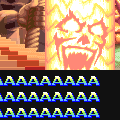•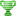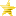• Loyal to the Game
Now that I look at the follow-up code, it does seem redundant... Removed.

Also thanks. Can't believe I missed that last one.

Later today I will do a quick update to the main archive and tell those who already downloaded it how to fix it. Updates uploaded. Gonna sleep for now.
Last Edit: February 07, 2009, 10:06:19 am by Jesuszilla